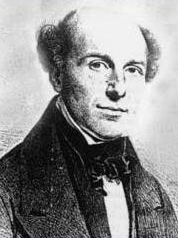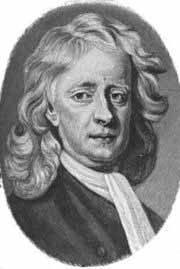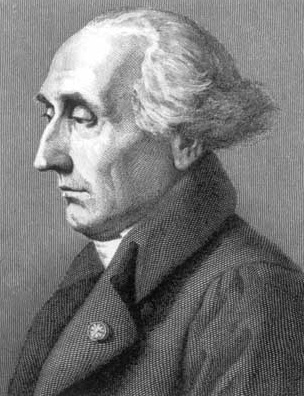PHYS 350 - 2016WHome Syllabus Problems## Syllabus

Below you will find the topics/concepts we have covered to date in the course as well as additional review materials that may be of assistance to you.

Please Read Through Chapters 1 - 4 and be familiar with all material therein.
We will cover most of these topics, which you have likely seen before, at a good pace.
Some ideas will be covered in more detail during class.
Review Notes for Topics in Chapters 1 - 4 of Taylor

01/04
Logistical Information. Motivation. Why Classical Mechanics?
What is Classical Mechanics? Newton, Lagrange, and Hamilton.
Basics: Time, Space, Frames, Mass, Force.
Review: Newton's First Law.  Newton's Second Law.  Newton's Third Law.
01/07 Introduction to Calculus of Variations: Shortest 2-Segment Path Between Points.
Shortest 3-Segment Path Between Points.
01/09 Shortest N+1-Segment Path Between Points. Example: A Random Selection of Paths.
Intro: Shortest Smooth Path Between Points.
01/11 Shortest Smooth Path Between Points. Functionals.
Calculus of Variations. General Functional of a Single Function. Euler-Lagrange Equations.
01/14 Kinetic Energy.  Work.  Conservative Forces. Central Forces. Potential Energy.  Total Energy.  Time-Dependent Potentials. Many Particle Energetics: Total Kinetic Energy, Total Potential Energy, Total Energy. Rigid Bodies.  The Virial Theorem
01/16 Euler-Lagrange Equations. Example: The Brachistocrone Problem.
01/18 Finding Euler-Lagrange when given a Functional. Functionals of Many Functions.
01/21 Calculus of Variations Applied to Mechanics.  The Lagrangian: A Functional for Newton's Laws. Hamilton's Principle of Least Action.  Lagrangian Mechanics.
01/23 Conservation of Momentum. Rocket Propulsion. Video: Robert Goddard Archival Footage. Video: SpaceX. Example:Launching a Rocket . The Centre of Mass Coordinate.
01/25 Lagrangian Mechanics.  The Pendulum.   Double Pendulum
01/28 Worked Solution: The Double Pendulum.  Quadratic Lagrangian.
01/30 Normal Modes of the Double Pendulum.  Example: Normal Modes The Double Pendulum.
02/01 Worked Solution: Two Masses and Three Springs
02/04 Point Grey Pendulum. Ignorable Coordinates. Noether's Theorem.
02/06 Noether's Theorem.  Translation Invariance: Momentum Conservation. Time-Translation Invariance: Hamiltonian is Constant. Atwood's Machine. Bead on a Rotating Wire.
02/08 Example: Bead on Rotating Wire
02/11 Group Project Meetings / In-Class: Springs in Space
02/13 Group Project Meetings / Kerbal Space Program
02/15 Motion of Two Particles under a Harmonic Force Law (Springs in Space).
The Effective Radial Potential for a 3D Harmonic Oscillator.  The Two-Body Problem in a Central Potential.  The General Effective Potential for the Two-Body Problem.  Kepler Problem.
02/18
02/20
02/22

Reading Week No Classes

 02/25 Two-Body Central Potential. Example: The Celestial Two Body Problem.  The Kepler Problem. 02/27 Midterm 03/01 The Kepler Problem: Solving the Radial Equation. 03/04 Worked Example: Practice Midterm; Geometry of Bound Solutions. Kepler's Second and Third Law. 03/06 Energy in the Kepler Problem.  Geometry of Unbound Solutions. Orbits in the Kepler Problem, Orbital Parameters. 03/08 Example: Changes of Orbit, Spaceship I 03/11 Example: Gravity Assist Maneuver 03/18 Rotational Motion of Rigid Bodies 03/20 Inertia Tensor, Examples: Sphere About Centre, Cylinder About Centre, Cone About Pivot 03/25 Euler Angles, Principle Moments, Principle Axes. Lagrangian for a Symmetric Top. 03/27 Normal Modes K and M Matrix Formalism 03/29 Hamiltonian Mechanics

 Home Syllabus Problems